Cyclotomic polynomials

circular polynomials

The polynomialsthat satisfy the relationwhere the product is taken over all positive divisorsof the number, includingitself. Over the field of complex numbers one has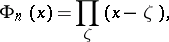where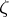ranges over the primitive-th roots of unity (cf. Primitive root). The degree ofis the number of integersamong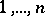that are relatively prime with. The polynomials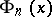can be computed recursively by dividing the polynomialby the product of all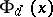,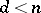,. The coefficients lie in the prime field; in case of characteristic zero, they are integers. Thus,If, moreover,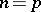is prime, thenThe polynomialcan be explicitly expressed using the Möbius function: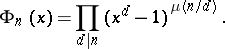For example,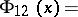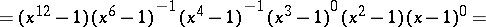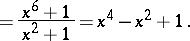All the polynomialsare irreducible over the field of rational numbers, but they may be reducible over finite prime fields. Thus, the relation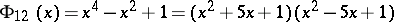is valid over the field of residues modulo 11.

The equation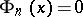, which gives all primitive-th roots of unity, is known as the equation of division of the circle. The solution of this equation in trigonometric form iswhere the fraction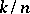is irreducible, i.e.andare relatively prime. The solution of the equation of division of the circle by radicals is closely connected with the problem of constructing a regular-gon, or with the equivalent problem of subdividing the circle intoequal parts; the latter problem can be solved using a straightedge and a pair of compasses if and only if the equationis solvable in quadratic radicals. It was shown by C.F. Gauss in 1801 that this condition is satisfied if and only if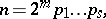whereis a non-negative integer and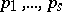are pairwise different prime numbers of the form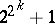, whereis a non-negative integer.